# Measuring Performance¶

Here’s some examples of how to find the optimal worker count and chunk size for different Dask operations. See the scripts in the github repository for samples of how these were measured.

Note

The first time a file is read can be quite slow if it’s not in cache. When running benchmarks make sure to load the file fully once (e.g. with data.mean().load()) before doing any timing.

import pandas
import numpy
import matplotlib.pyplot as plt


## ERA5 single level one year mean¶

Lets look at performance for reading in a year of ERA5 single level data. This data comes from compressed netcdf files, so the time spent decompressing will affect our measurements.

I’ve run for various chunk sizes and Dask cluster sizes

path = "/g/data/rt52/era5/single-levels/reanalysis/2t/2001/2t_era5_oper_sfc_*.nc"
with xarray.open_mfdataset(
path, combine="nested", concat_dim="time", chunks=chunks
) as ds:
var = ds[variable]

start = time.perf_counter()
duration = time.perf_counter() - start


and saved the results in a netcdf file

df = pandas.read_csv('read_speed/era5_t2_load.csv')
df

duration data_size chunk_size time latitude longitude workers threads
0 38.058817 36379929600 194987520 NaN 182 360 4 1
1 38.475415 36379929600 97493760 NaN 182 180 4 1
2 38.962101 36379929600 48746880 NaN 91 180 4 1
3 39.011292 36379929600 97493760 NaN 91 360 4 1
4 50.406360 36379929600 6093360 93.0 91 180 4 1
... ... ... ... ... ... ... ... ...
112 17.296662 36379929600 6093360 93.0 91 180 16 1
113 18.460571 36379929600 97493760 NaN 182 180 16 1
114 18.579301 36379929600 97493760 NaN 91 360 16 1
115 20.263248 36379929600 194987520 NaN 182 360 16 1
116 20.702859 36379929600 389975040 NaN 182 720 16 1

117 rows × 8 columns

To start off with, let’s look at the mean walltime for a given cluster and chunk size. I’ll focus on a single thread as well.

mean = df.groupby(['workers','chunk_size','threads']).mean().reset_index()


and to narrow the results down further I’ll select a single chunk layout of {'time': None, 'latitude': 91, 'longitude': 180}. This is the file chunk size in the horizontal dimensions, about 49 MB per chunk.

df_91_180 = one_thread[(one_thread.latitude==91) & (one_thread.longitude==180) & numpy.isnan(one_thread.time)]
df_91_180 = df_91_180.set_index('workers', drop=False)
df_91_180

workers chunk_size threads duration data_size time latitude longitude
workers
1 1 48746880 1 192.943019 3.637993e+10 NaN 91.0 180.0
2 2 48746880 1 86.172158 3.637993e+10 NaN 91.0 180.0
4 4 48746880 1 39.269419 3.637993e+10 NaN 91.0 180.0
8 8 48746880 1 21.874852 3.637993e+10 NaN 91.0 180.0
16 16 48746880 1 13.159739 3.637993e+10 NaN 91.0 180.0
32 32 48746880 1 7.959964 3.637993e+10 NaN 91.0 180.0
48 48 48746880 1 6.344361 3.637993e+10 NaN 91.0 180.0

Plotting the walltime taken for different worker sizes looks good, ideally this should be proportional to $$1 / \textrm{workers}$$.

Note

The maximum number of CPUs on a single Gadi node is 48, and the default Dask cluster can’t go beyond that.

wall = (df_91_180['duration'])
wall.plot()
plt.ylabel('walltime (s)');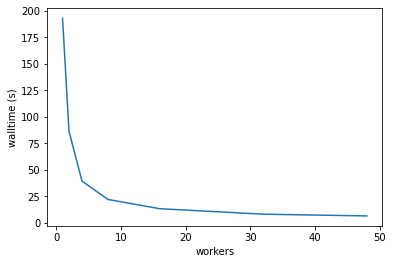Gadi’s run costs are proportional to $$\textrm{walltime}\times\textrm{workers}$$. The cost minimum is at about 4 workers.

Note

Remember this is the cost for just loading the data and calculating the global mean. Further processing can affect the cost scaling as well

cost = (df_91_180['duration'] * df_91_180['workers']/60/60*2)
cost.plot(color='tab:orange')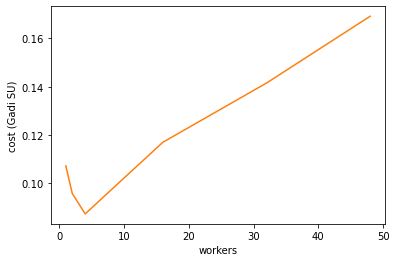To find a good balance of walltime and cost we want to minimise $$\textrm{walltime}\times\textrm{cost}$$. The best worker count by this metric for this single operation is 32 workers.

(wall * cost).plot(color='tab:green')
plt.ylim([0,2.5])
plt.ylabel('scaling metric (lower is better)');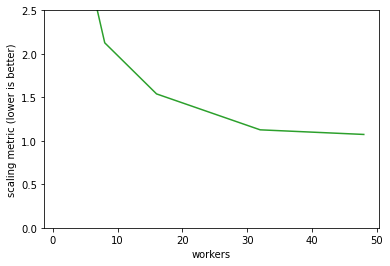We’ve worked out the best worker count to use, how about the chunk size? The best size here is in the 50 to 100 MB range. Generally the chunk size should be an integer multiple of the file chunk size to make things easy for the NetCDF library, but this doesn’t have a huge effect.

one_thread[one_thread.workers == 32].plot('chunk_size','duration', legend=False)

xlabels = ['0 MB', '100 MB', '200 MB', '300 MB', '400 MB']
plt.xticks([dask.utils.parse_bytes(x) for x in xlabels], labels=xlabels)

plt.xlabel('chunk size');
plt.ylabel('walltime (s)');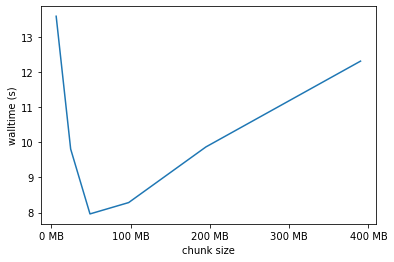def result_scaling(df):
wall = df['duration']
cost = df['duration'] * df['workers'] / 60 / 60 * 2
scaling = wall * cost
return wall, cost, scaling

def plot_results(csv, target_workers=32):

df_91_180 = df_91_180.set_index('workers', drop=False)

wall, cost, scaling = result_scaling(df_91_180)

ax = plt.subplot(2,3,1)
wall.plot(ax=ax, color='tab:blue')
plt.ylabel('walltime (s)');

ax = plt.subplot(2,3,2)
cost.plot(color='tab:orange')

ax = plt.subplot(2,3,3)
scaling.plot(color='tab:green')
#plt.ylim([0,1200])
plt.ylabel('scaling metric (lower is better)');

wall, cost, scaling = result_scaling(df_chunksize)

xlabels = ['0 MB', '100 MB', '200 MB', '300 MB']

ax = plt.subplot(2,3,4)
wall.plot(ax=ax, color='tab:blue')
plt.xticks([dask.utils.parse_bytes(x) for x in xlabels], labels=xlabels)
plt.xlabel('chunk size');
plt.ylabel('walltime (s)');

ax = plt.subplot(2,3,5)
cost.plot(ax=ax, color='tab:orange')
plt.xticks([dask.utils.parse_bytes(x) for x in xlabels], labels=xlabels)
plt.xlabel('chunk size');

ax = plt.subplot(2,3,6)
scaling.plot(ax=ax, color='tab:green')
plt.xticks([dask.utils.parse_bytes(x) for x in xlabels], labels=xlabels)
plt.xlabel('chunk size');
plt.ylabel('scaling metric (lower is better)');

def optimal_size(csv):

cost = mean['duration']*mean['duration']*mean['workers']
opt = mean.iloc[cost.sort_values().index]
print(f'Workers:  {opt.workers:2.0f}')
print(f"Walltime:  {pandas.Timedelta(opt.duration, 's').round('s')}")
print(f"Cost:     {(opt.duration/60/60)**2*opt.workers*2:5.2f} SU")
print(f"Chunks:   {opt[['latitude', 'longitude']].to_dict()}")


## ERA5 10-year climatology¶

Calculating a daily mean climatology from 10 years of hourly ERA5 data, using climtas functions for resampling and groupby, saving the results to a compressed netcdf file.

path = "/g/data/rt52/era5/single-levels/reanalysis/2t/200*/2t_era5_oper_sfc_*.nc"
var = 't2m'

with xarray.open_mfdataset(
path, combine="nested", concat_dim="time", chunks=chunks
) as ds:
var = ds[variable]
if isel is not None:
var = var.isel(**isel)

out = os.path.join(os.environ['TMPDIR'],'sample.nc')

start = time.perf_counter()
daily = climtas.blocked_resample(var, time=24).mean()
climatology = climtas.blocked_groupby(daily, time='dayofyear').mean()
climtas.io.to_netcdf_throttled(climatology, out)
duration = time.perf_counter() - start


plt.figure(figsize=(20,10))

Workers: 48.0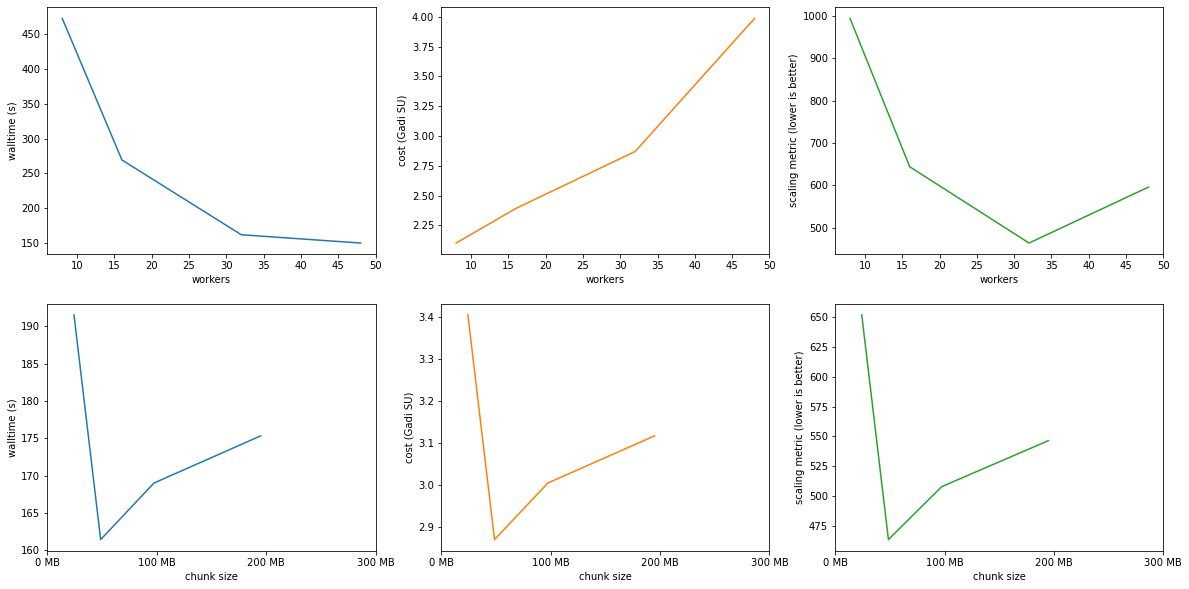### Optimal results:¶

optimal_size('read_speed/era5_t2_climatology.csv')

Workers:  32